# How to Use the Calculation field (Expert and Ultimate tariffs)

The Calculation field is a hidden field that is not displayed on the published form. The field allows you to calculate a value based on the data that your respondents enter into different fields on your form. The result of the field is always a number.

To display the result of the Calculation field, you need to use the piping function (@) in the Text form fields. One form can have multiple Calculation fields.

## What is the difference between the Calculation field and the Formula field?

The Formula field only works with numbers! The field allows you to set a mathematical expression that will use numbers that your respondents enter in other fields on your form. Read more about the Formula field

The Calculation field allows you to calculate a value based on the conditions that you set for other fields. For example, you have a multiple-choice field with Option 1 and Option 2. If your respondent chooses Option 1, the Calculation adds 1 to the total, if your respondent chooses Option 2, the Calculation adds 10 to the total.

The result values of the Calculation field can be used in the Formula field and vice versa.

## The Calculation field allows you to:

1. Create multiple-choice and picture-choice quizzes that calculate multiple scores based on the chosen options. Read more
2. Create website calculators with conditional calculations. For example, to calculate a monthly payment based on different conditions.
3. Create order forms that calculate the total prices based on the options chosen.

## How to use the Calculation field

### Example 1: a form with one Calculation field

Your form has a multiple-choice field ‘A village or a city?’ and if the answer is village, the Lifestyle calculation adds 1; if the answer is city, the Lifestyle calculation adds 2.

1. Go to Form Elements -> Hidden and add the Calculation field to any part of your form.

2. Enter the field name.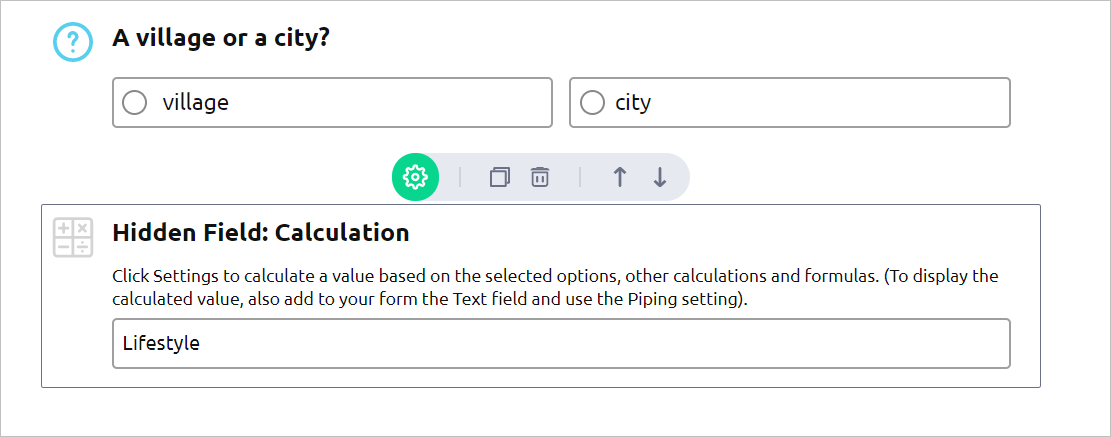3. Open the settings section. Set an initial value for the field, if required, and click the Add Calculation button. You will get the When - Then condition fields.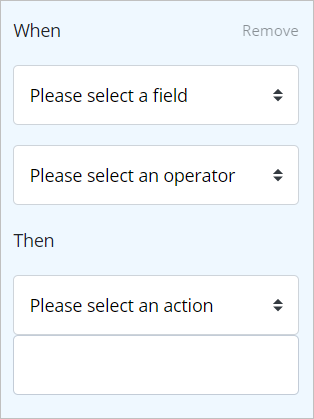4. Use drop-down menus to choose available options and set up the first condition:

When the field ‘A village or a city?’
contains: village

Add and set up the second condition:

When the field ‘A village or a city?’
contains: city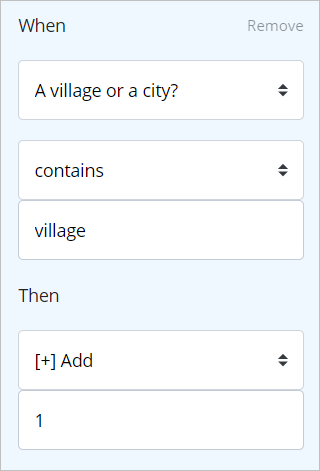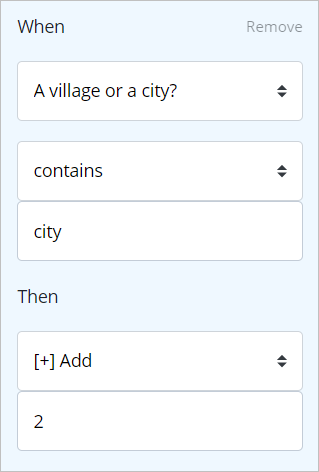5. To display the calculation result on your form, Add the Text field to your form. Type in the text you would like to display for your respondents and use the @ function to reference the Calculation field result.

### Example 2: a form with multiple Calculation fields

Your form has a multiple-choice field: ‘Do you like skyscrapers?’ and if the answer is yes, the calculation ‘New York’ adds 1; if the answer is no, the calculation ‘Paris’ adds 1.

1. Add two Calculation fields to your form. Name the fields ‘New York’ and ‘Paris’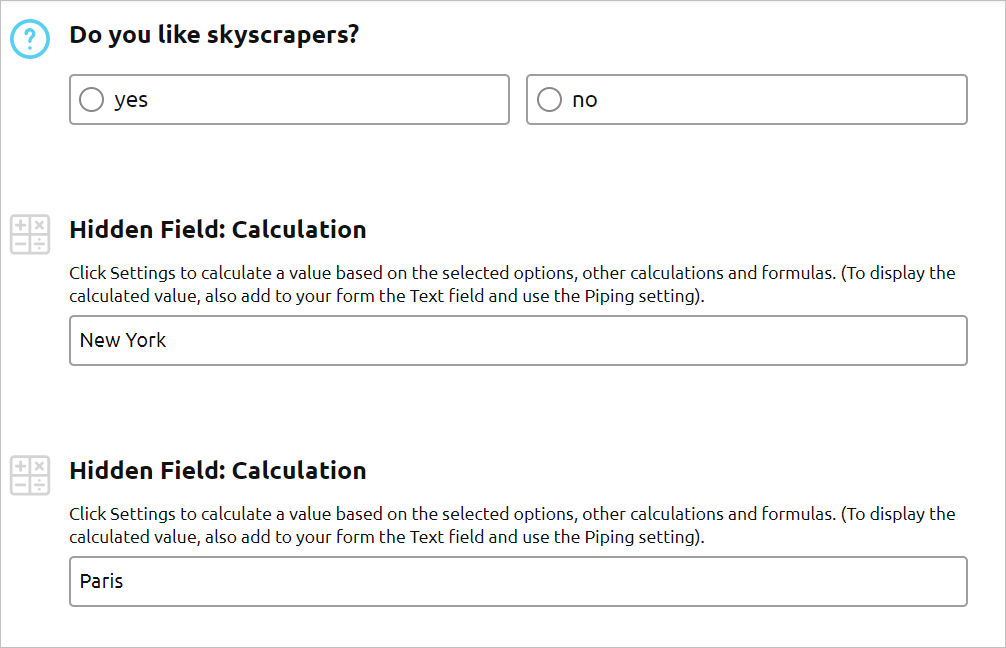2. Open the settings for the New York calculation field and complete the set up:

When the field ‘Do you like skyscrapers?’
contains: yes